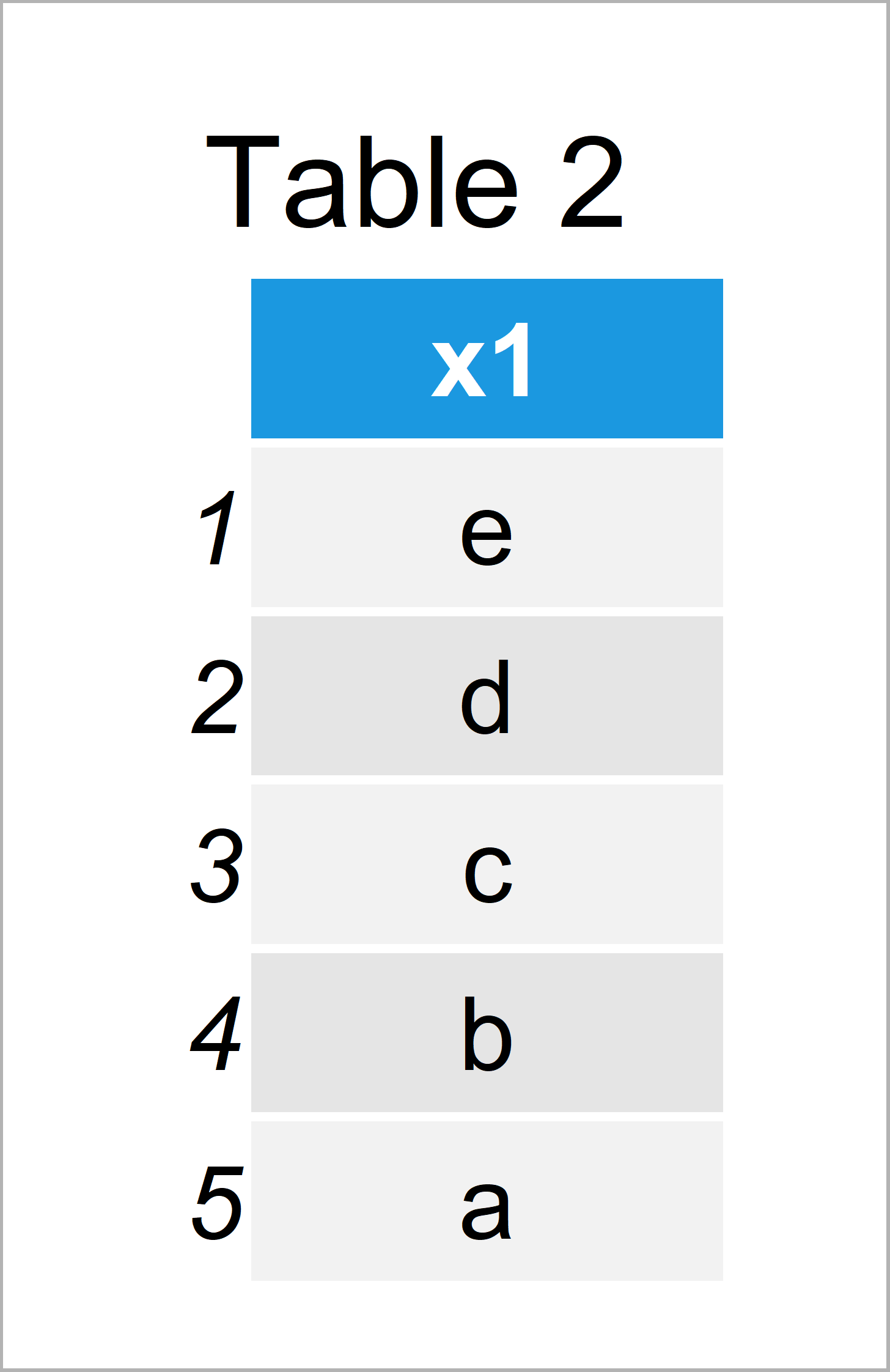# Extract Single Column as Data Frame in R (3 Examples)

This tutorial shows how to select a single variable from a data frame and keep the data.frame class in R programming.

The post contains three examples for the manipulation of data frames. To be more precise, the post will consist of these contents:

Let’s dive into it…

## Creating Example Data

The following data will be used as basement for this R tutorial:

```data <- data.frame(x1 = letters[5:1],    # Create example data
x2 = "x",
x3 = 1:5)
data                                     # Print example data```Table 1 shows that our example data contains five rows and the three columns x1, x2, and x3.

## Example 1: Extract Single Variable as Vector (The Problem)

Example 1 replicates the problem that the R programming language typically converts single data frame columns to vectors.

Usually, we would extract a single data frame column using the \$ operator

```data\$x1                                  # Extract single column using \$
#  "e" "d" "c" "b" "a"```

…or square brackets and a comma:

```data[ , 1]                               # Extract single column using [ , ]
#  "e" "d" "c" "b" "a"```

In most cases this is fine. However, what if we want to retain the data.frame class when we are extracting only one variable from a data frame? That’s what I’ll explain in the next examples…

## Example 2: Extract Single Variable as Data Frame Using Square Brackets

This example illustrates how to use square brackets to select only one column as a data frame.

For this, we simply have to avoid the comma between the square brackets:

`data                                  # Single column as data frame using []`Table 2 shows the output of the previous syntax – A data frame containing only the variable x1.

## Example 3: Extract Single Variable as Data Frame Using drop Argument

Another alternative when we want to keep the data frame class is provided by the drop argument.

We can specify drop to be equal to FALSE in case we do not want to convert our column to a vector object:

`data[ , 1, drop = FALSE]                 # Single column as data frame using drop`Table 3 shows the output of the previous code – A data frame containing exactly the same values as in Example 2, but this time created with the drop argument.

## Video & Further Resources

I have recently released a video on my YouTube channel, which explains the R codes of this tutorial. You can find the video tutorial below.

Please accept YouTube cookies to play this video. By accepting you will be accessing content from YouTube, a service provided by an external third party.If you accept this notice, your choice will be saved and the page will refresh.

In addition, you might have a look at the related tutorials of statisticsglobe.com.

Summary: At this point you should have learned how to extract one variable as data frame and how to print this column with row names to the RStudio console using the R programming language. Tell me about it in the comments, in case you have any further questions.

Subscribe to the Statistics Globe Newsletter